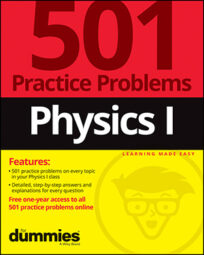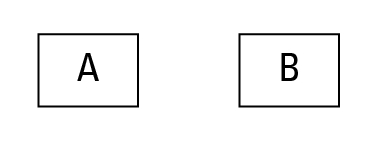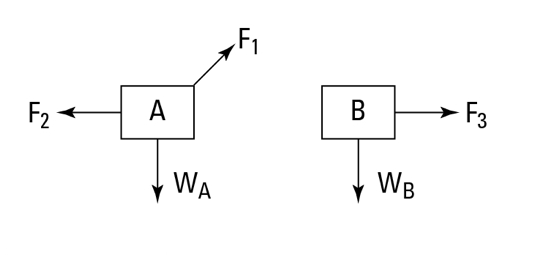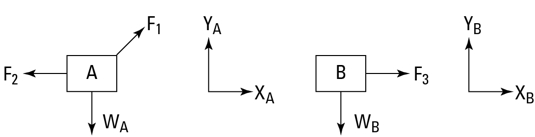##### Physics I: 501 Practice Problems For Dummies (+ Free Online Practice)In physics, force problems typically ask you to predict what will happen when you apply force to an object, and usually there’s no handy illustration to help you visualize what’s being described. Fortunately, you can create your own diagram so you can better picture what a question is asking you. Follow this seven-step method to solve force problems:

1. Draw each of the objects you’re interested in.

Here’s an example:2. Identify the forces acting on each object.

For each force acting on one of the objects from Step 1, draw an arrow that indicates the direction of the force, as shown in the following figure. Note that the tail of the arrow indicates which part of the object the force is acting on.3. Draw a free-body diagram for each object.

If you’re following this step-by-step guide, you’ve already drawn a free-body diagram. It’s listed separately because it’s the most important step!

4. Choose a coordinate system for each object.

Usually you draw the x-direction horizontally and the y-direction vertically, as shown in the following figure. However, when dealing with inclined planes, sometimes you want to choose your coordinate axes parallel and perpendicular to the plane.5. For each object, write down each component of Newton’s second law.

For angular motion problems, choose an axis of rotation and write down the angular version of Newton’s second law.

6. Include any constraints.

Sometimes you have more variables than equations at this point. Write down any other information you know. For example, if a car is driving on a flat road, you know that the vertical component of its acceleration is zero.

7. Solve the equations.

Now that you’ve used all your physics knowledge, all you have to do is the algebra.